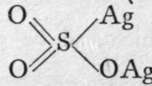When sulphur, selenium, or tellurium is heated in air, the element takes fire and burns ; the chief product in each case is the dioxide. That of sulphur is a colourless gas, possessing the well-known odour of burning sulphur; it is condensable to a liquid at -8°, and it freezes at -79°. The gas is soluble in water, uniting with it to form the acid, H2SO3 ; on cooling the solution to -6° and saturating it with the gas, crystals of the formula H2SO3.8H2O separate; this is a hydrate of sulphurous acid. Selenious anhydride, a white solid, also dissolves in water, and from the solution selenious acid crystallises out, with the formula H2SeO3. It would naturally be imagined that these acids should have the structural formulas OS=(OH)2 and 0=Se(OH)2, inasmuch as the oxides are 0=S=0 and 0=Se=0 ; moreover, the chlorides 0=S=C12 and 0=Se=Cl2, thionyl and selenosyl chlorides, are known; and these react at once with water, forming the acids. It is to be presumed that there is exchange of chlorine for hydroxyl, as usual: 0=S=C12 + 2HOH = 0=S=(0H)2 + 2HC1. But there is evidence, similar in kind to that adduced in- the case of nitrous and phosphorous acids, to show that while sodium sulphite has the formula 0=S=(ONa)2, silver sulphite is better expressed by, sulphur being a hexad.r